#> Warning: This tutorial was written with Giotto version 0.3.6.9046, your version
#> is 1.0.3.This is a more recent version and results should be reproducible
library(Giotto)

# 1. set working directory
results_folder = '/path/to/directory/'

# 2. set giotto python path
# set python path to your preferred python version path
# set python path to NULL if you want to automatically install (only the 1st time) and use the giotto miniconda environment
python_path = NULL
if(is.null(python_path)) {
installGiottoEnvironment()
}

## Dataset explanation

10X genomics recently launched a new platform to obtain spatial expression data using a Visium Spatial Gene Expression slide.

The Visium kidney data to run this tutorial can be found here

Visium technology:High resolution png from original tissue: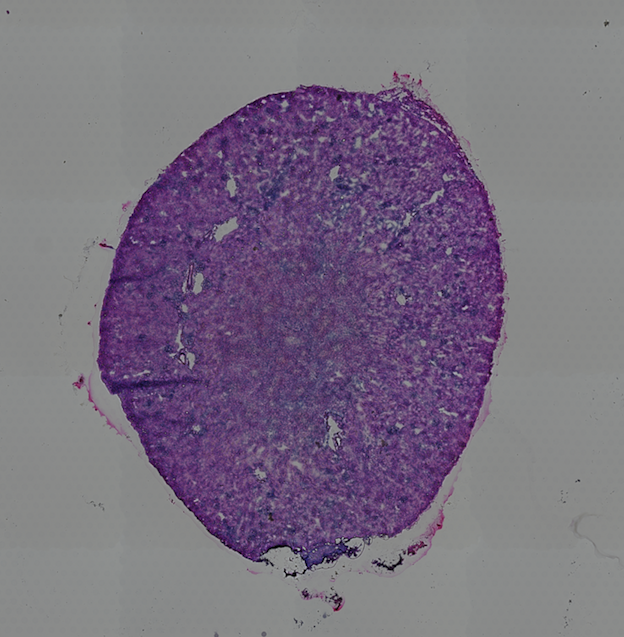## Part 1: Giotto global instructions and preparations

## create instructions
instrs = createGiottoInstructions(save_dir = results_folder,
save_plot = TRUE,
show_plot = FALSE,
python_path = python_path)

## provide path to visium folder
data_path = '/path/to/Kidney_data/'

## part 2: Create Giotto object & process data

## directly from visium folder
visium_kidney = createGiottoVisiumObject(visium_dir = data_path, expr_data = 'raw',
png_name = 'tissue_lowres_image.png',
gene_column_index = 2, instructions = instrs)

## update and align background image
# problem: image is not perfectly aligned
spatPlot(gobject = visium_kidney, cell_color = 'in_tissue', show_image = T, point_alpha = 0.7,
save_param = list(save_name = '2_a_spatplot_image'))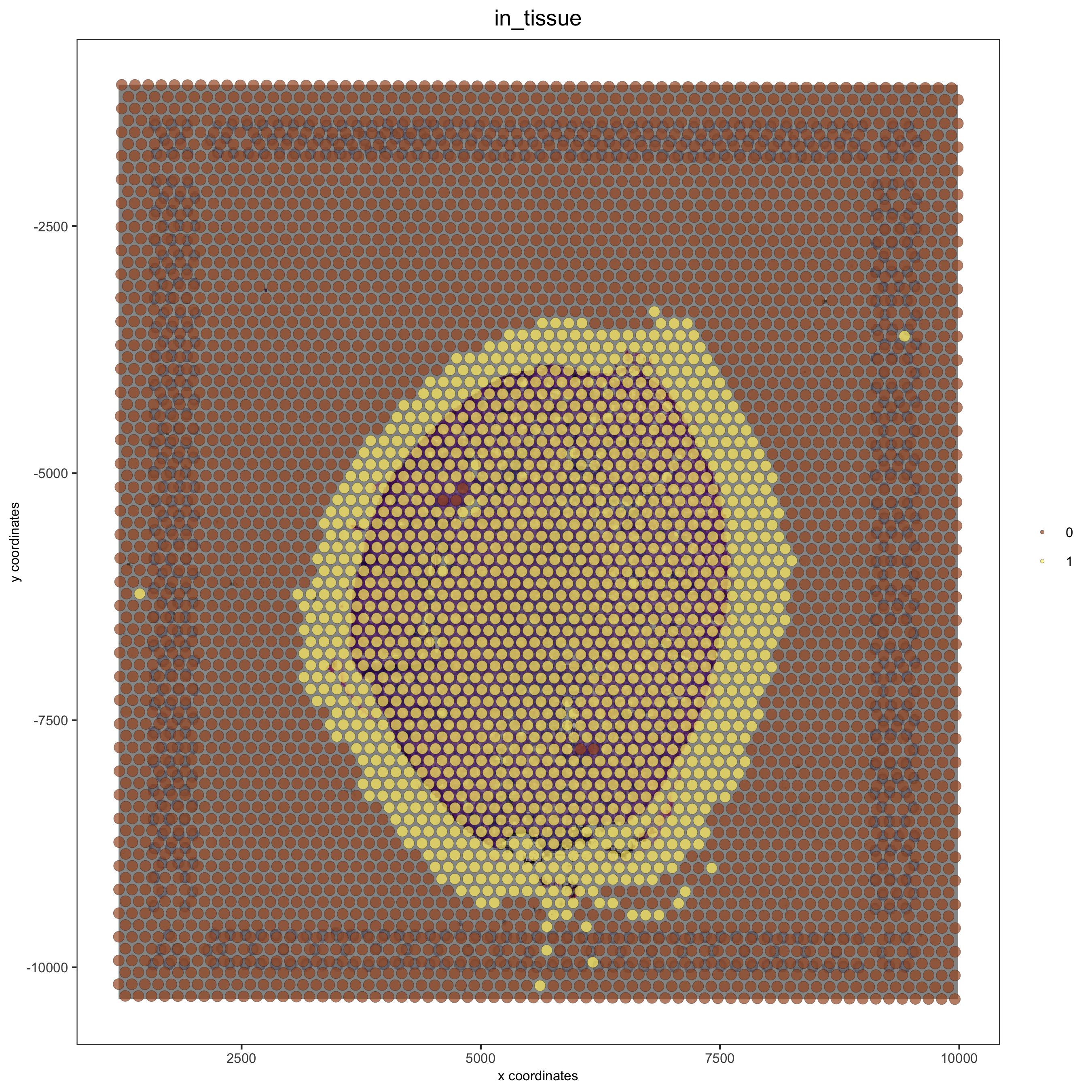# check name
showGiottoImageNames(visium_kidney) # "image" is the default name
# adjust parameters to align image (iterative approach)
visium_kidney = updateGiottoImage(visium_kidney, image_name = 'image',

# now it's aligned
spatPlot(gobject = visium_kidney, cell_color = 'in_tissue', show_image = T, point_alpha = 0.7,
save_param = list(save_name = '2_b_spatplot_image_adjusted'))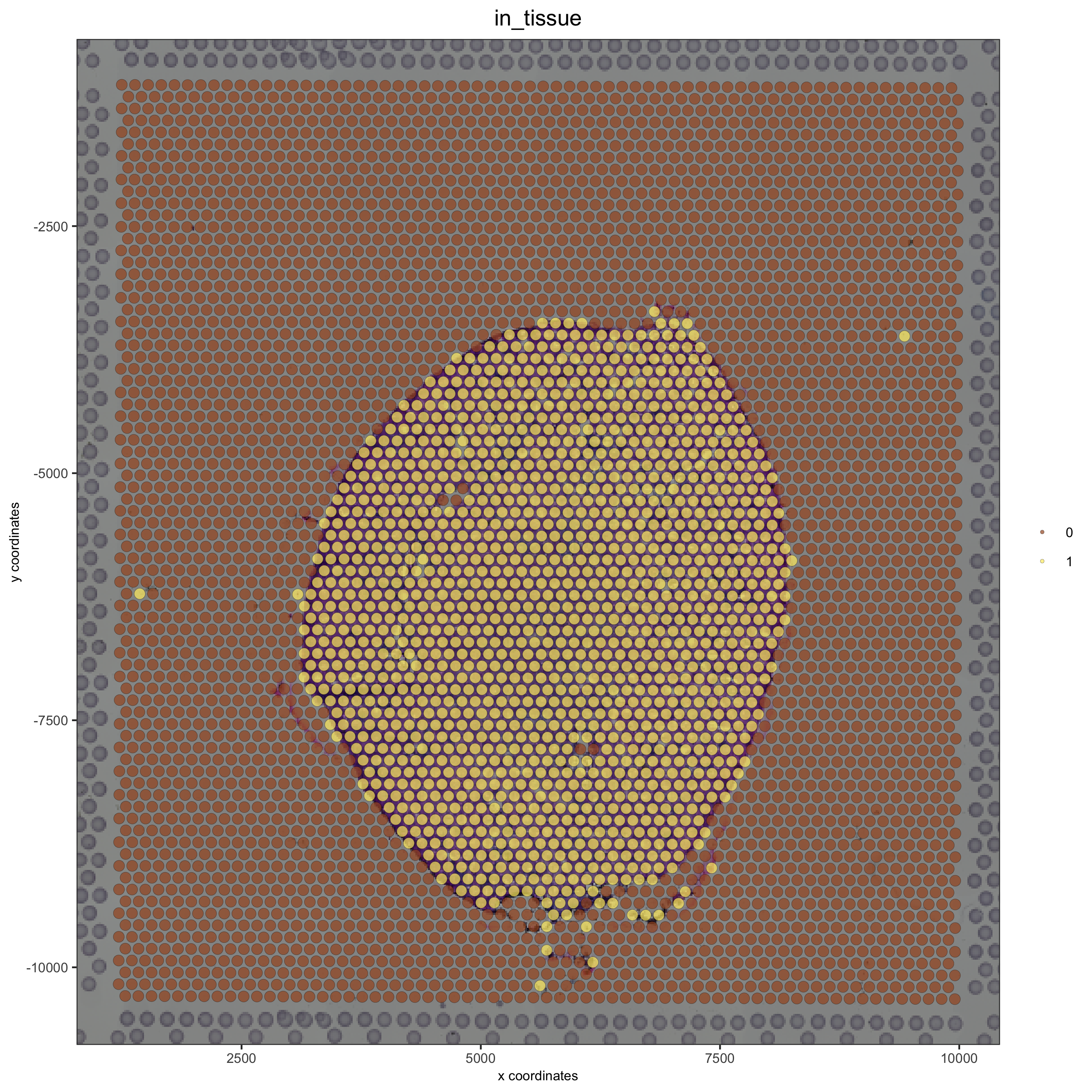## check metadata

## compare in tissue with provided jpg
spatPlot(gobject = visium_kidney, cell_color = 'in_tissue', point_size = 2,
cell_color_code = c('0' = 'lightgrey', '1' = 'blue'),
save_param = list(save_name = '2_c_in_tissue'))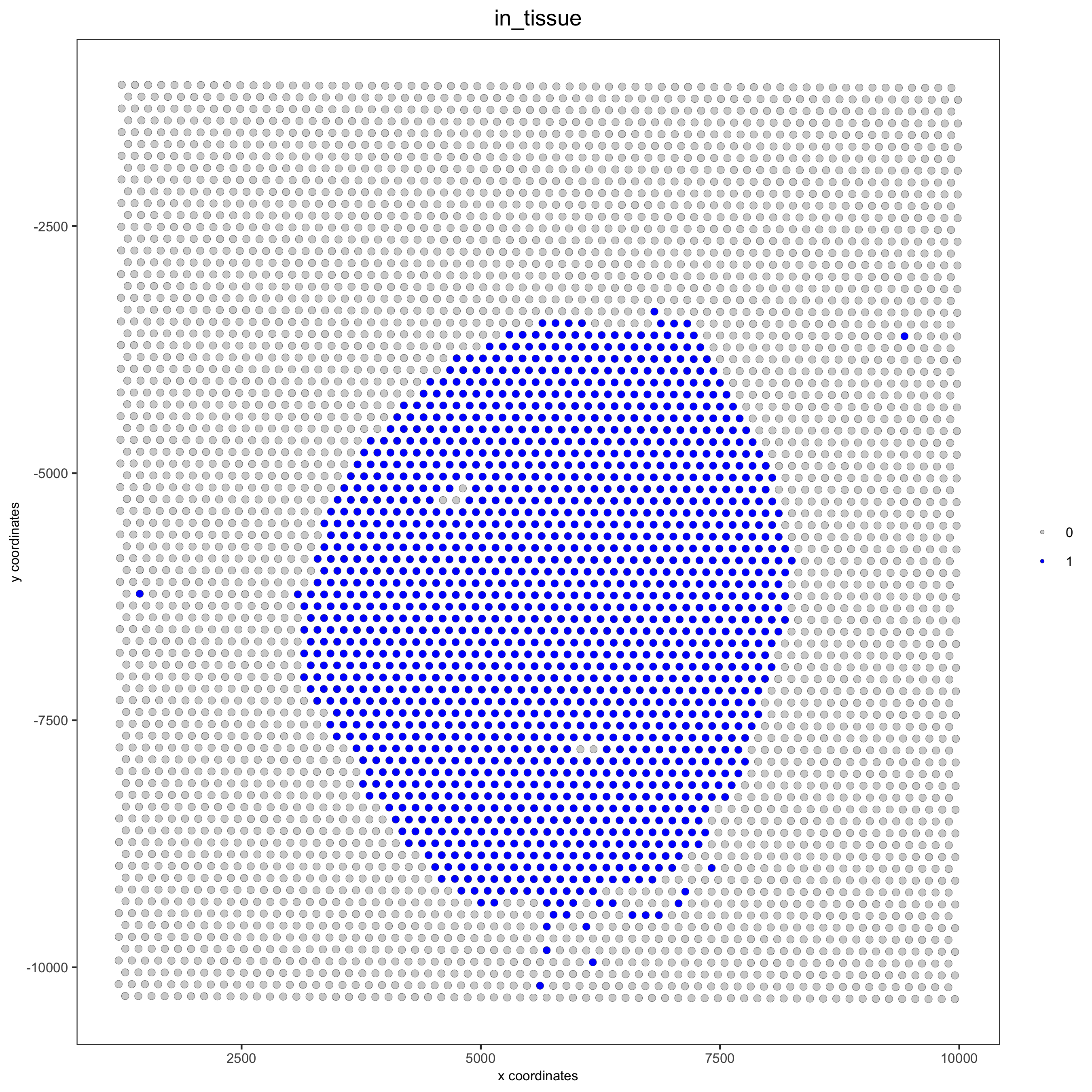## subset on spots that were covered by tissue
in_tissue_barcodes = metadata[in_tissue == 1]$cell_ID visium_kidney = subsetGiotto(visium_kidney, cell_ids = in_tissue_barcodes) ## filter visium_kidney <- filterGiotto(gobject = visium_kidney, expression_threshold = 1, gene_det_in_min_cells = 50, min_det_genes_per_cell = 1000, expression_values = c('raw'), verbose = T) ## normalize visium_kidney <- normalizeGiotto(gobject = visium_kidney, scalefactor = 6000, verbose = T) ## add gene & cell statistics visium_kidney <- addStatistics(gobject = visium_kidney) ## visualize spatPlot2D(gobject = visium_kidney, show_image = T, point_alpha = 0.7, save_param = list(save_name = '2_d_spatial_locations'))spatPlot2D(gobject = visium_kidney, show_image = T, point_alpha = 0.7, cell_color = 'nr_genes', color_as_factor = F, save_param = list(save_name = '2_e_nr_genes'))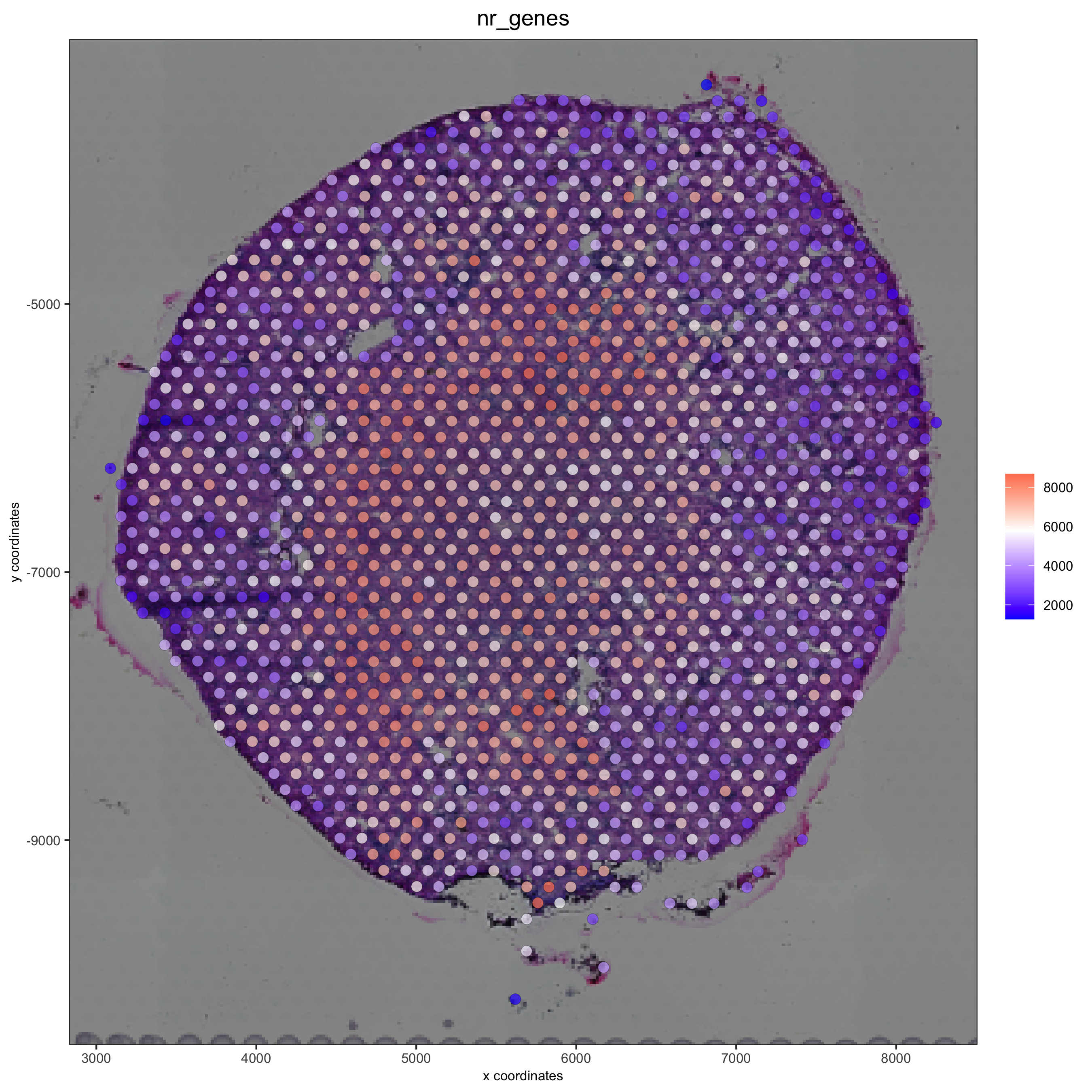## part 3: dimension reduction ## highly variable genes (HVG) visium_kidney <- calculateHVG(gobject = visium_kidney, save_param = list(save_name = '3_a_HVGplot'))## run PCA on expression values (default) visium_kidney <- runPCA(gobject = visium_kidney, center = TRUE, scale_unit = TRUE) screePlot(visium_kidney, ncp = 30, save_param = list(save_name = '3_b_screeplot'))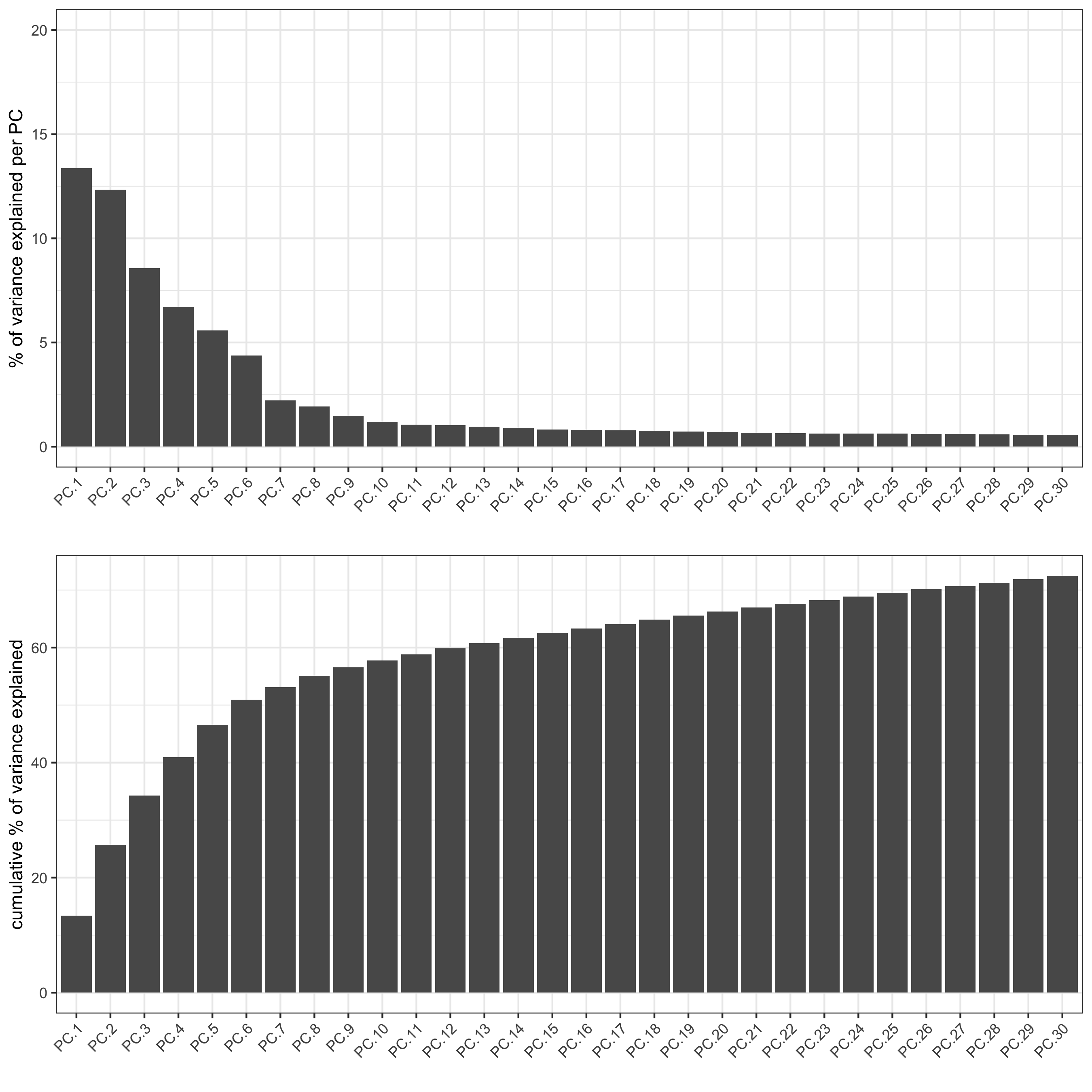plotPCA(gobject = visium_kidney, save_param = list(save_name = '3_c_PCA_reduction'))## run UMAP and tSNE on PCA space (default) visium_kidney <- runUMAP(visium_kidney, dimensions_to_use = 1:10) plotUMAP(gobject = visium_kidney, save_param = list(save_name = '3_d_UMAP_reduction'))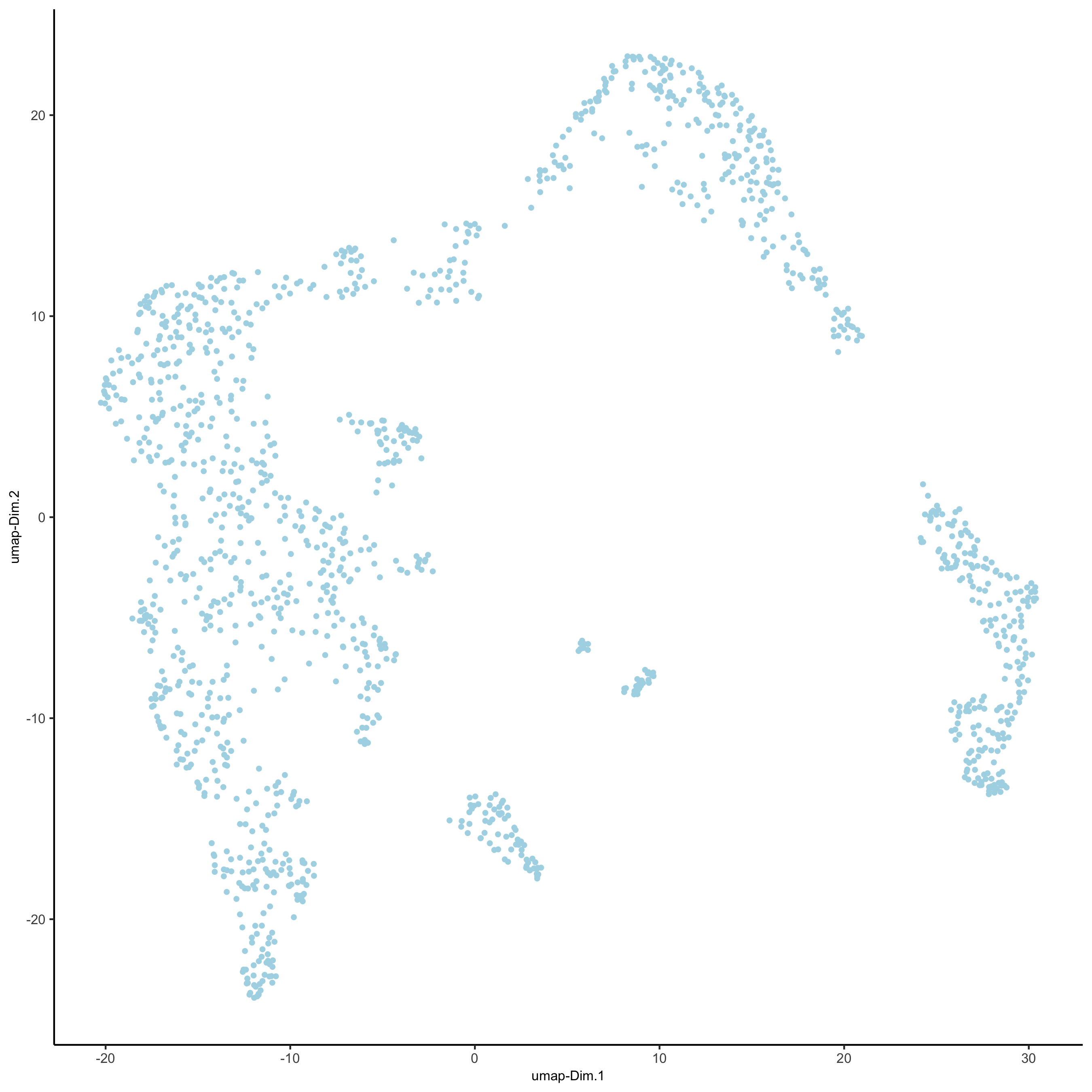visium_kidney <- runtSNE(visium_kidney, dimensions_to_use = 1:10) plotTSNE(gobject = visium_kidney, save_param = list(save_name = '3_e_tSNE_reduction'))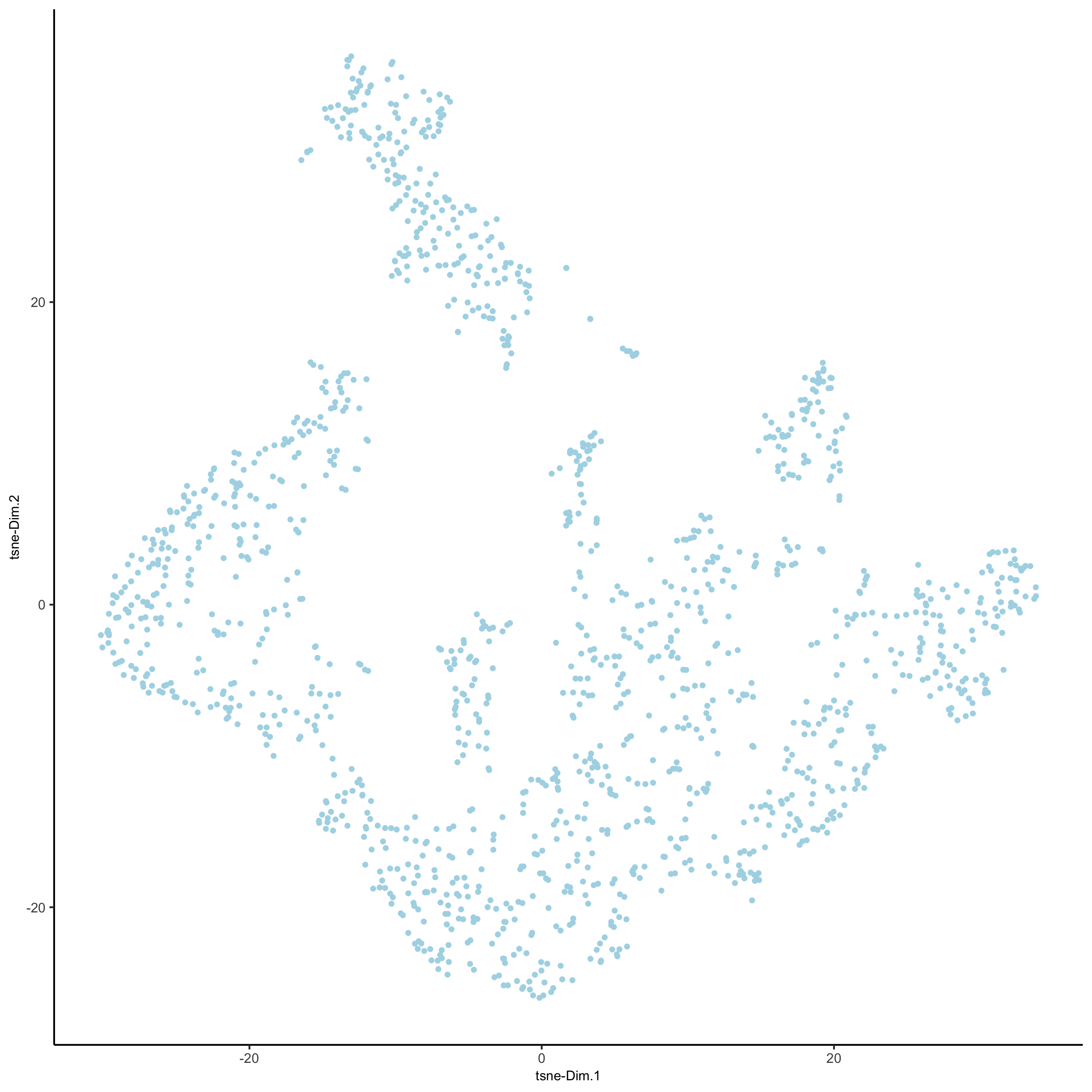## part 4: cluster ## sNN network (default) visium_kidney <- createNearestNetwork(gobject = visium_kidney, dimensions_to_use = 1:10, k = 15) ## Leiden clustering visium_kidney <- doLeidenCluster(gobject = visium_kidney, resolution = 0.4, n_iterations = 1000) plotUMAP(gobject = visium_kidney, cell_color = 'leiden_clus', show_NN_network = T, point_size = 2.5, save_param = list(save_name = '4_a_UMAP_leiden'))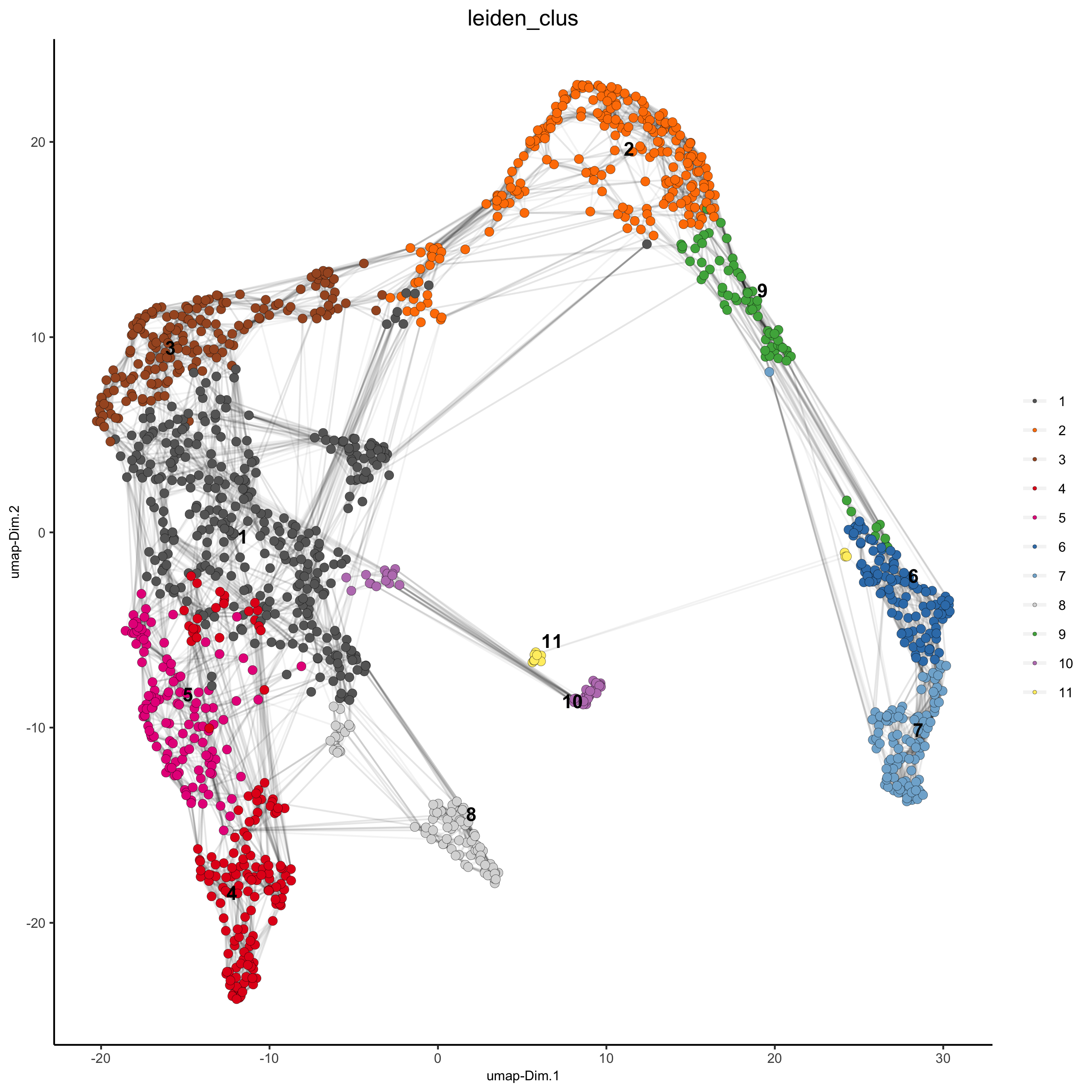## part 5: co-visualize # expression and spatial spatDimPlot(gobject = visium_kidney, cell_color = 'leiden_clus', dim_point_size = 2, spat_point_size = 2.5, save_param = list(save_name = '5_a_covis_leiden'))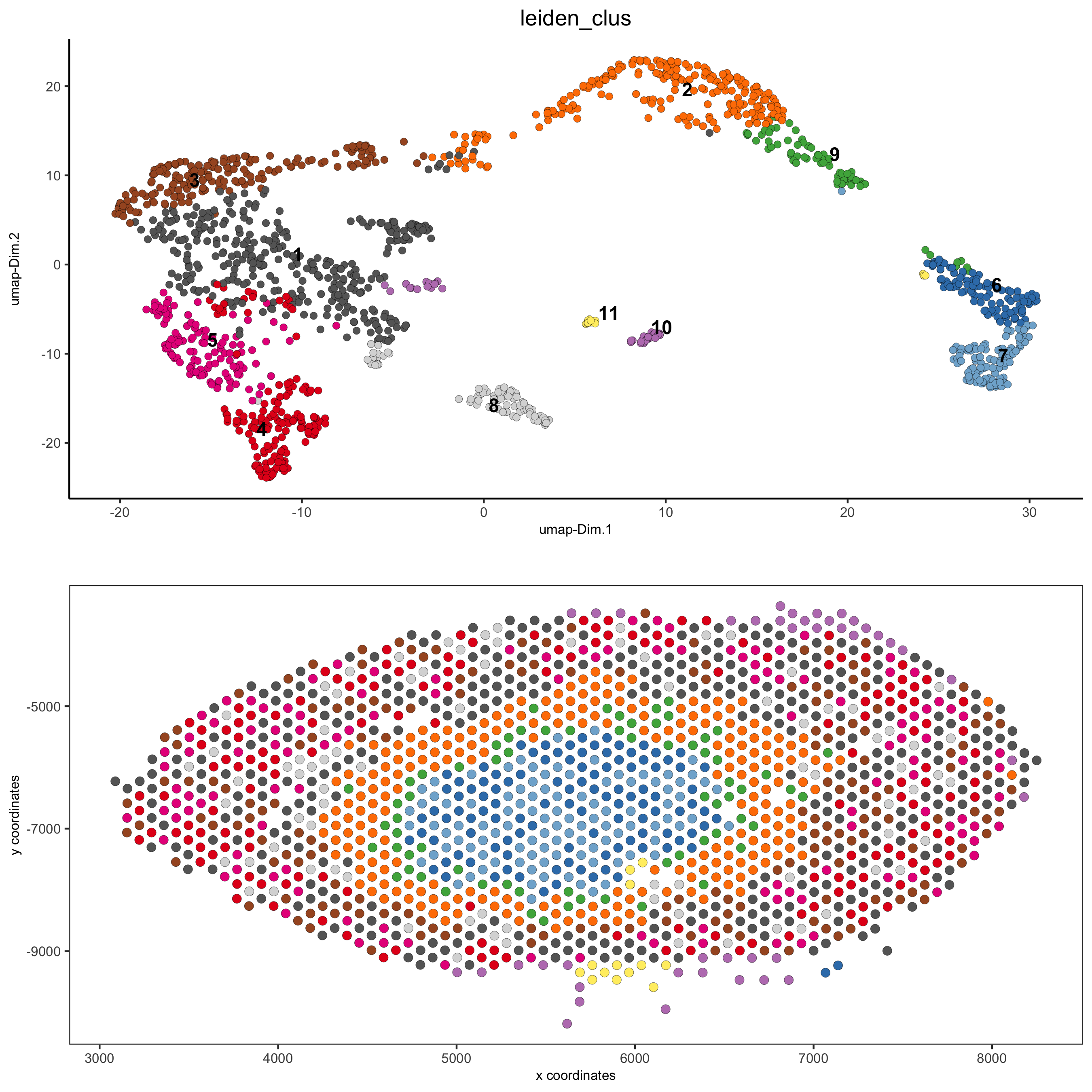spatDimPlot(gobject = visium_kidney, cell_color = 'nr_genes', color_as_factor = F, dim_point_size = 2, spat_point_size = 2.5, save_param = list(save_name = '5_b_nr_genes'))## part 6: cell type marker gene detection #### gini gini_markers_subclusters = findMarkers_one_vs_all(gobject = visium_kidney, method = 'gini', expression_values = 'normalized', cluster_column = 'leiden_clus', min_genes = 20, min_expr_gini_score = 0.5, min_det_gini_score = 0.5) topgenes_gini = gini_markers_subclusters[, head(.SD, 2), by = 'cluster']$genes

# violinplot
violinPlot(visium_kidney, genes = unique(topgenes_gini), cluster_column = 'leiden_clus',
strip_text = 8, strip_position = 'right',
save_param = c(save_name = '6_a_violinplot_gini', base_width = 5, base_height = 10))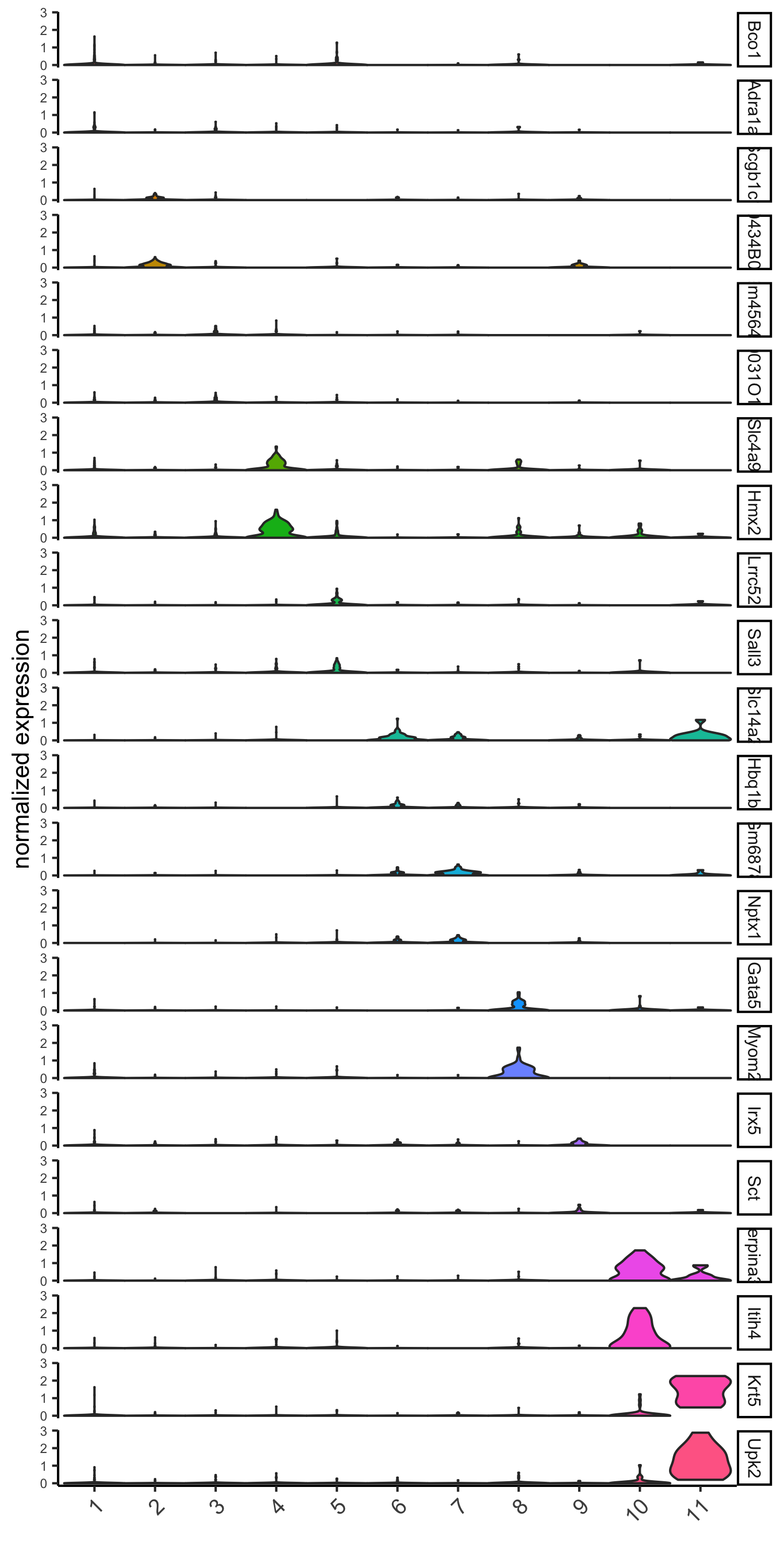# cluster heatmap
x_text_size = 10, y_text_size = 10,
save_param = c(save_name = '6_b_metaheatmap_gini'))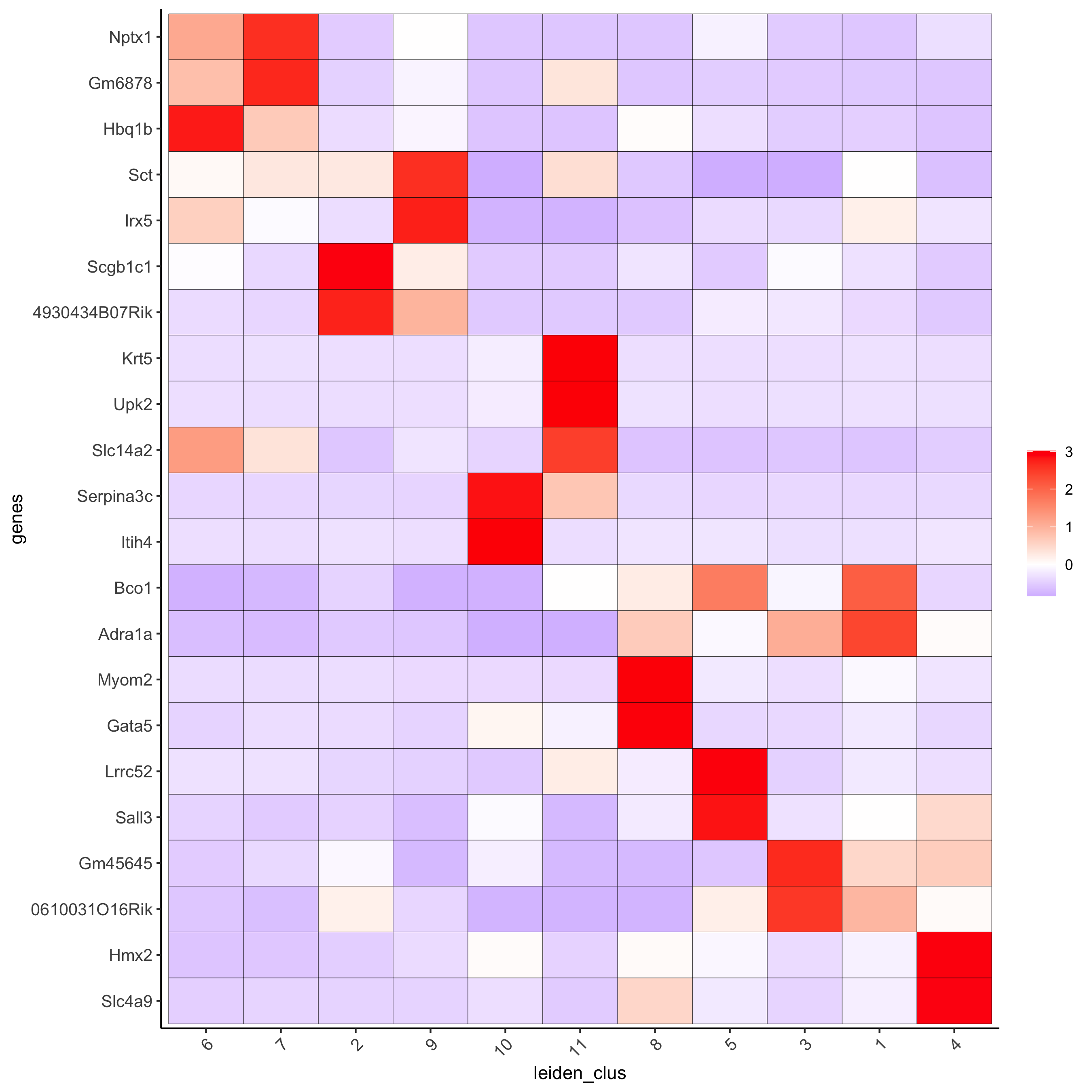# umap plots
dimGenePlot2D(visium_kidney, expression_values = 'scaled',
genes = gini_markers_subclusters[, head(.SD, 1), by = 'cluster']$genes, cow_n_col = 3, point_size = 1, save_param = c(save_name = '6_c_gini_umap', base_width = 8, base_height = 5))#### scran scran_markers_subclusters = findMarkers_one_vs_all(gobject = visium_kidney, method = 'scran', expression_values = 'normalized', cluster_column = 'leiden_clus') topgenes_scran = scran_markers_subclusters[, head(.SD, 2), by = 'cluster']$genes

# violinplot
violinPlot(visium_kidney, genes = unique(topgenes_scran), cluster_column = 'leiden_clus',
strip_text = 10, strip_position = 'right',
save_param = c(save_name = '6_d_violinplot_scran', base_width = 5))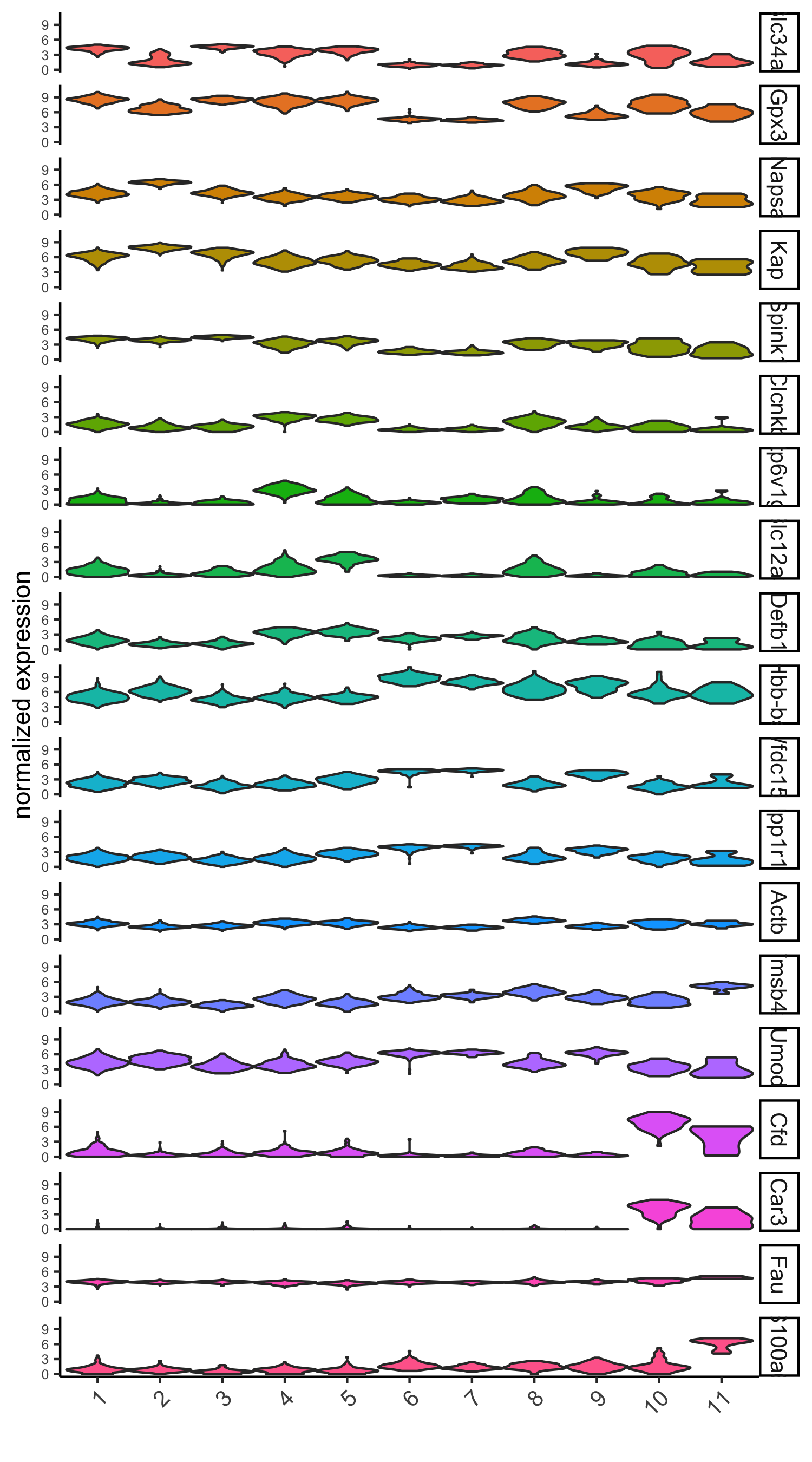# cluster heatmap
save_param = c(save_name = '6_e_metaheatmap_scran'))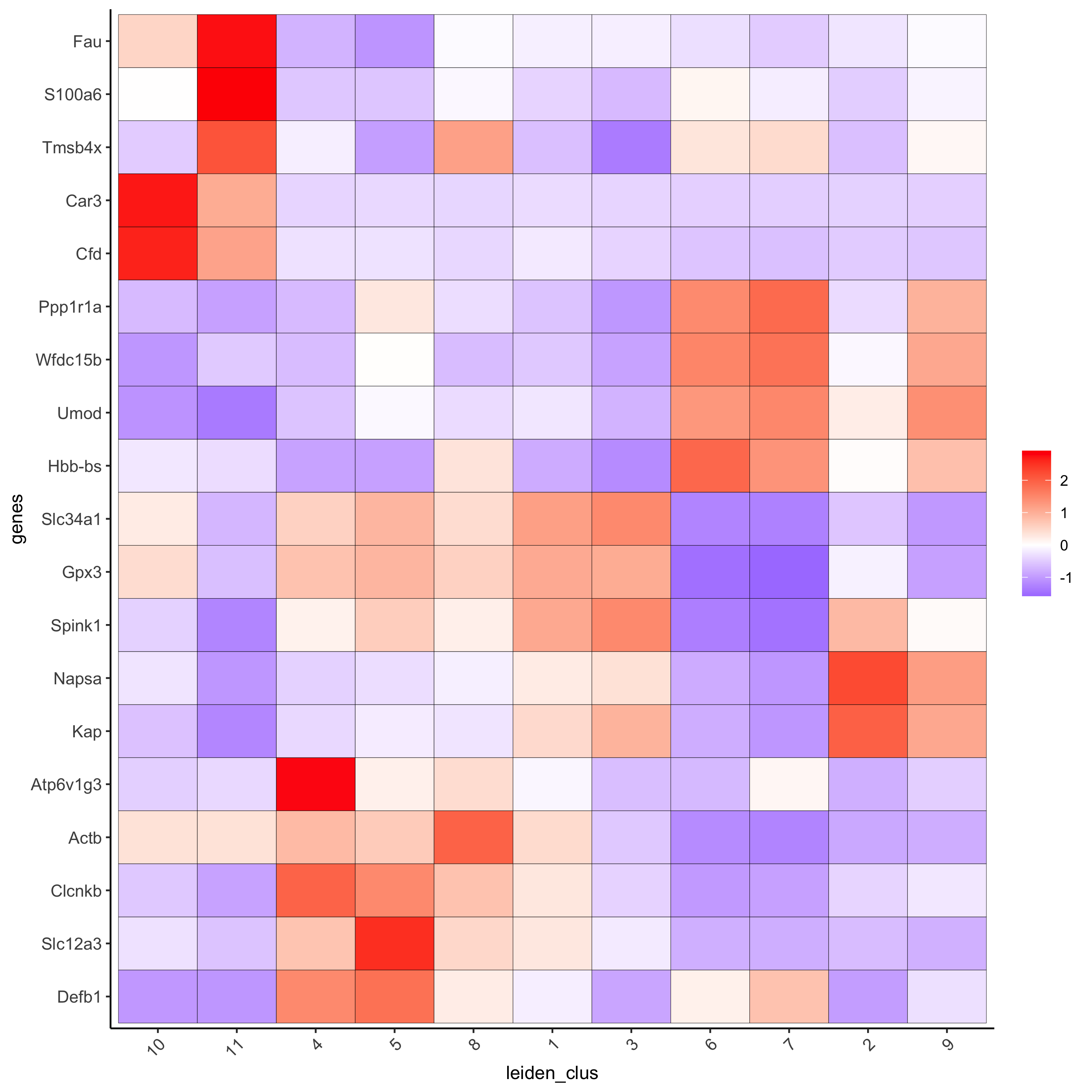# umap plots
dimGenePlot(visium_kidney, expression_values = 'scaled',
genes = scran_markers_subclusters[, head(.SD, 1), by = 'cluster']$genes, cow_n_col = 3, point_size = 1, save_param = c(save_name = '6_f_scran_umap', base_width = 8, base_height = 5))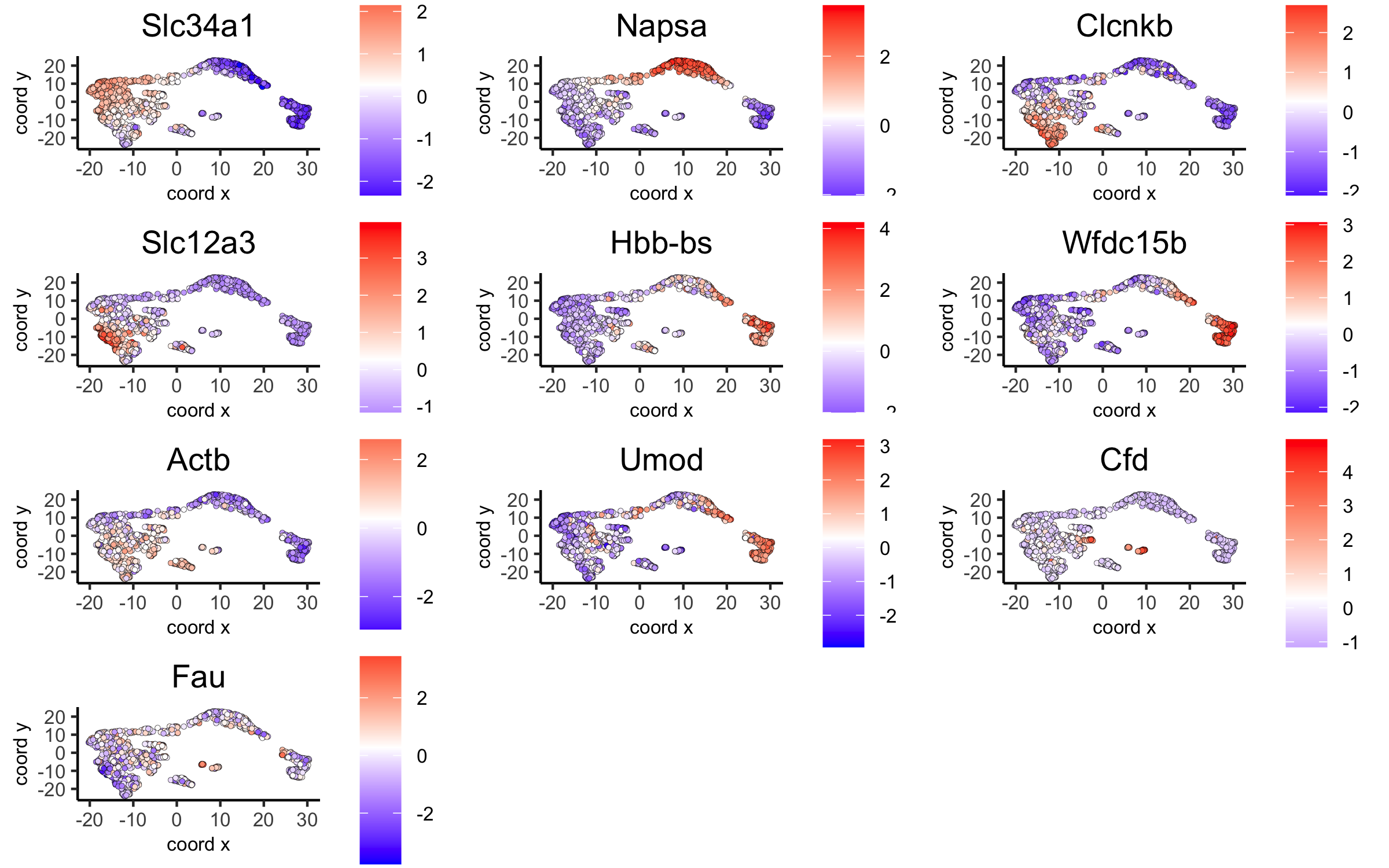## part 7: cell-type annotation Visium spatial transcriptomics does not provide single-cell resolution, making cell type annotation a harder problem. Giotto provides 3 ways to calculate enrichment of specific cell-type signature gene list: - PAGE - rank - hypergeometric test See the mouse Visium brain dataset for an example. ## part 8: spatial grid visium_kidney <- createSpatialGrid(gobject = visium_kidney, sdimx_stepsize = 400, sdimy_stepsize = 400, minimum_padding = 0) spatPlot(visium_kidney, cell_color = 'leiden_clus', show_grid = T, grid_color = 'red', spatial_grid_name = 'spatial_grid', save_param = c(save_name = '8_grid'))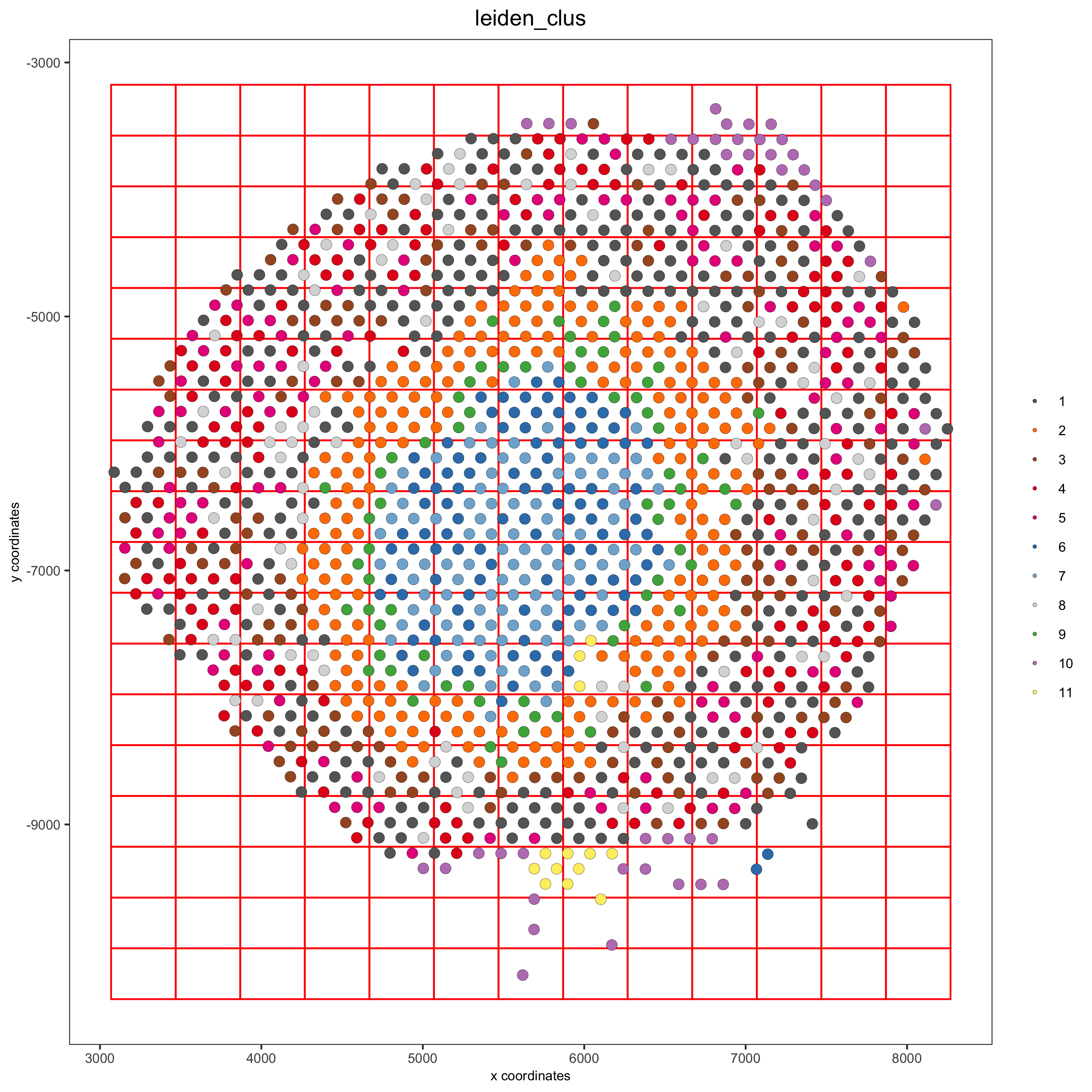## part 9: spatial network ## delaunay network: stats + creation plotStatDelaunayNetwork(gobject = visium_kidney, maximum_distance = 400, save_param = c(save_name = '9_a_delaunay_network'))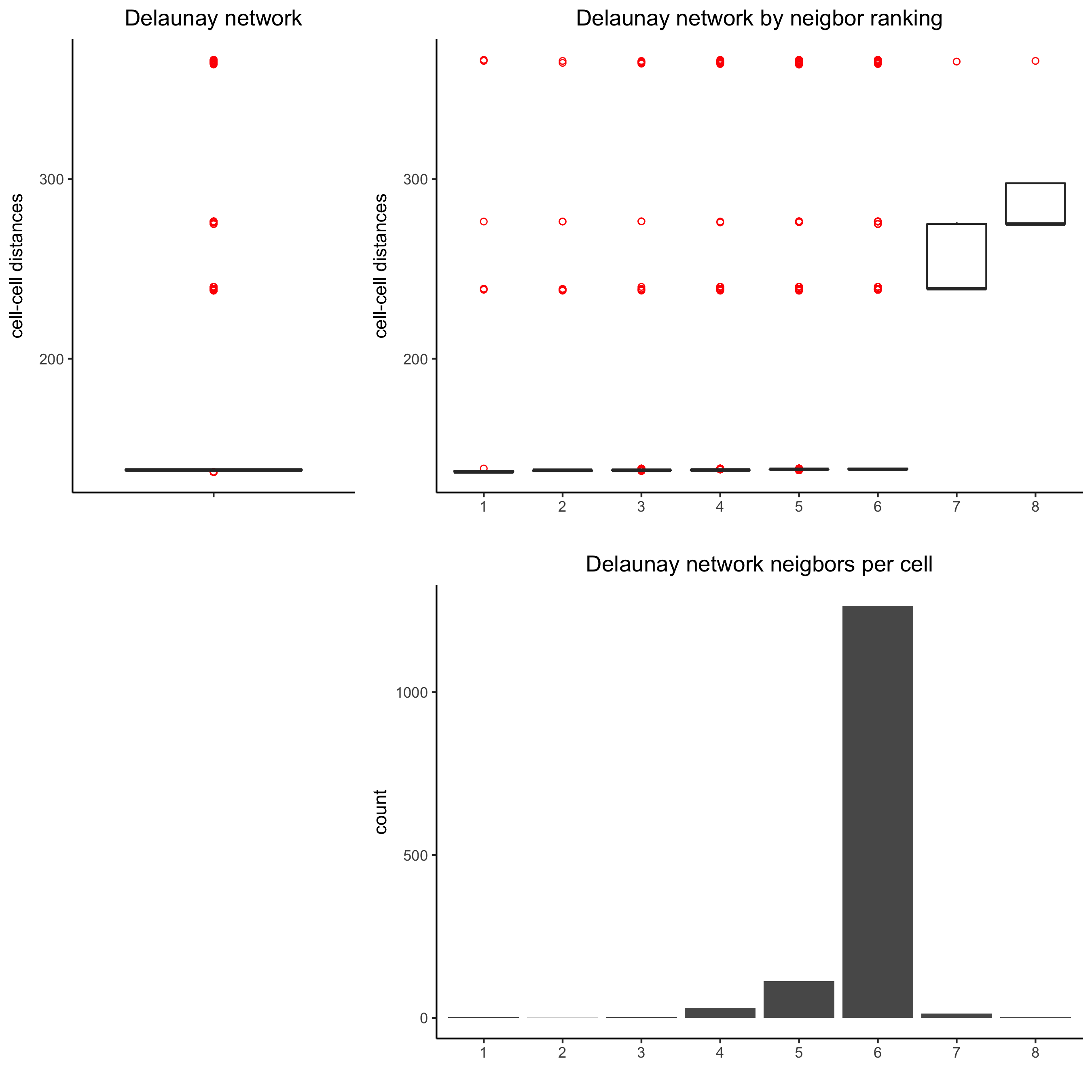visium_kidney = createSpatialNetwork(gobject = visium_kidney, minimum_k = 0) showNetworks(visium_kidney) spatPlot(gobject = visium_kidney, show_network = T, network_color = 'blue', spatial_network_name = 'Delaunay_network', save_param = c(save_name = '9_b_delaunay_network'))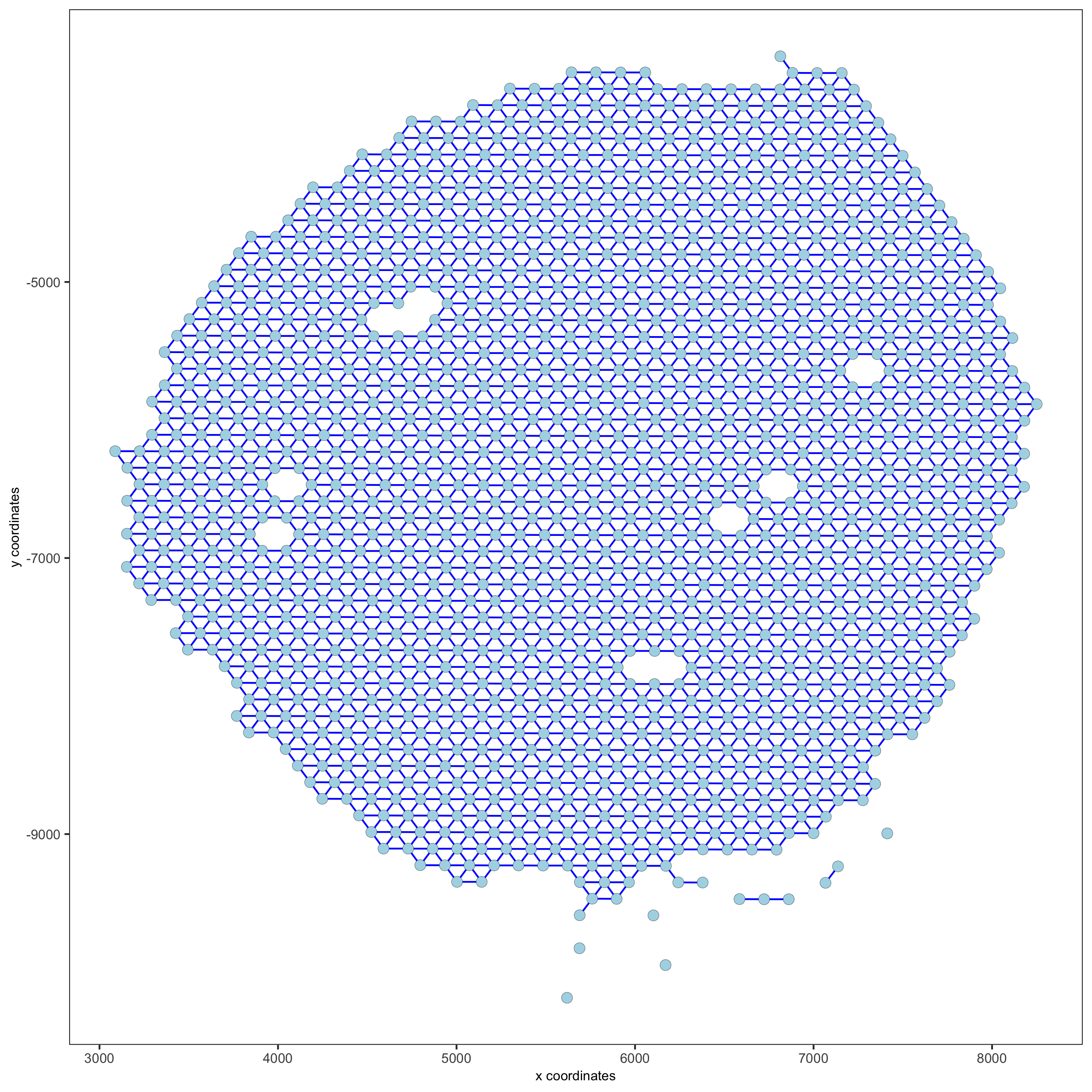## part 10: spatial genes ### Spatial genes ## kmeans binarization kmtest = binSpect(visium_kidney) spatGenePlot(visium_kidney, expression_values = 'scaled', genes = kmtest$genes[1:6], cow_n_col = 2, point_size = 1.5,
save_param = c(save_name = '10_a_spatial_genes_km'))## rank binarization
ranktest = binSpect(visium_kidney, bin_method = 'rank')
spatGenePlot(visium_kidney, expression_values = 'scaled',
genes = ranktest$genes[1:6], cow_n_col = 2, point_size = 1.5, save_param = c(save_name = '10_b_spatial_genes_rank'))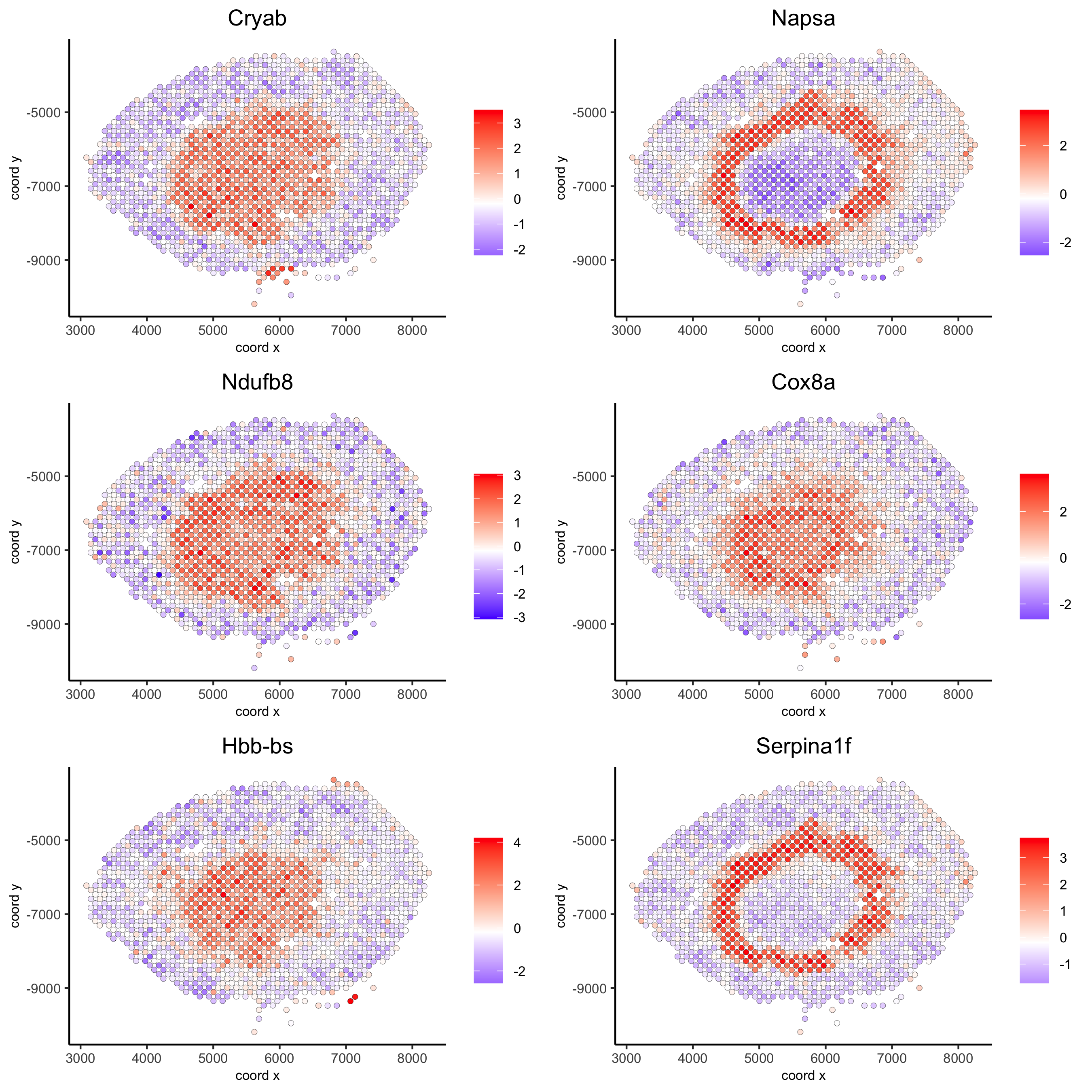### Spatial co-expression patterns ## spatially correlated genes ## ext_spatial_genes = kmtest[1:500]$genes

# 1. calculate gene spatial correlation and single-cell correlation
# create spatial correlation object
spat_cor_netw_DT = detectSpatialCorGenes(visium_kidney,
method = 'network',
spatial_network_name = 'Delaunay_network',
subset_genes = ext_spatial_genes)

# 2. identify most similar spatially correlated genes for one gene
Napsa_top10_genes = showSpatialCorGenes(spat_cor_netw_DT, genes = 'Napsa', show_top_genes = 10)
spatGenePlot(visium_kidney, expression_values = 'scaled',
genes = c('Napsa', 'Kap', 'Defb29', 'Prdx1'), point_size = 3,
save_param = c(save_name = '10_d_Napsa_correlated_genes'))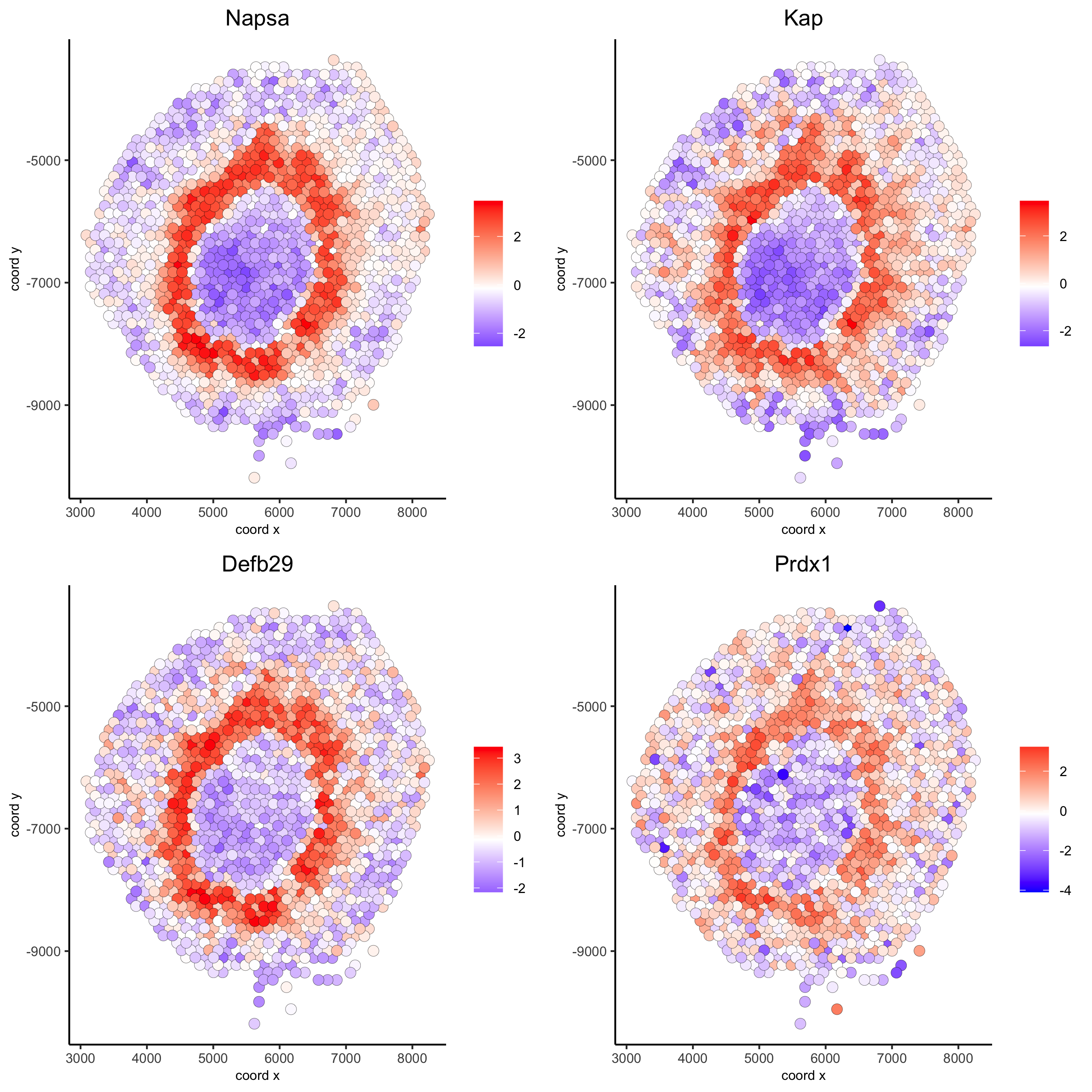# 3. cluster correlated genes & visualize
spat_cor_netw_DT = clusterSpatialCorGenes(spat_cor_netw_DT, name = 'spat_netw_clus', k = 8)

heatmSpatialCorGenes(visium_kidney, spatCorObject = spat_cor_netw_DT, use_clus_name = 'spat_netw_clus',
save_param = c(save_name = '10_e_heatmap_correlated_genes', save_format = 'pdf',
base_height = 6, base_width = 8, units = 'cm'),
heatmap_legend_param = list(title = NULL))# 4. rank spatial correlated clusters and show genes for selected clusters
netw_ranks = rankSpatialCorGroups(visium_kidney, spatCorObject = spat_cor_netw_DT, use_clus_name = 'spat_netw_clus',
save_param = c(save_name = '10_f_rank_correlated_groups',
base_height = 3, base_width = 5))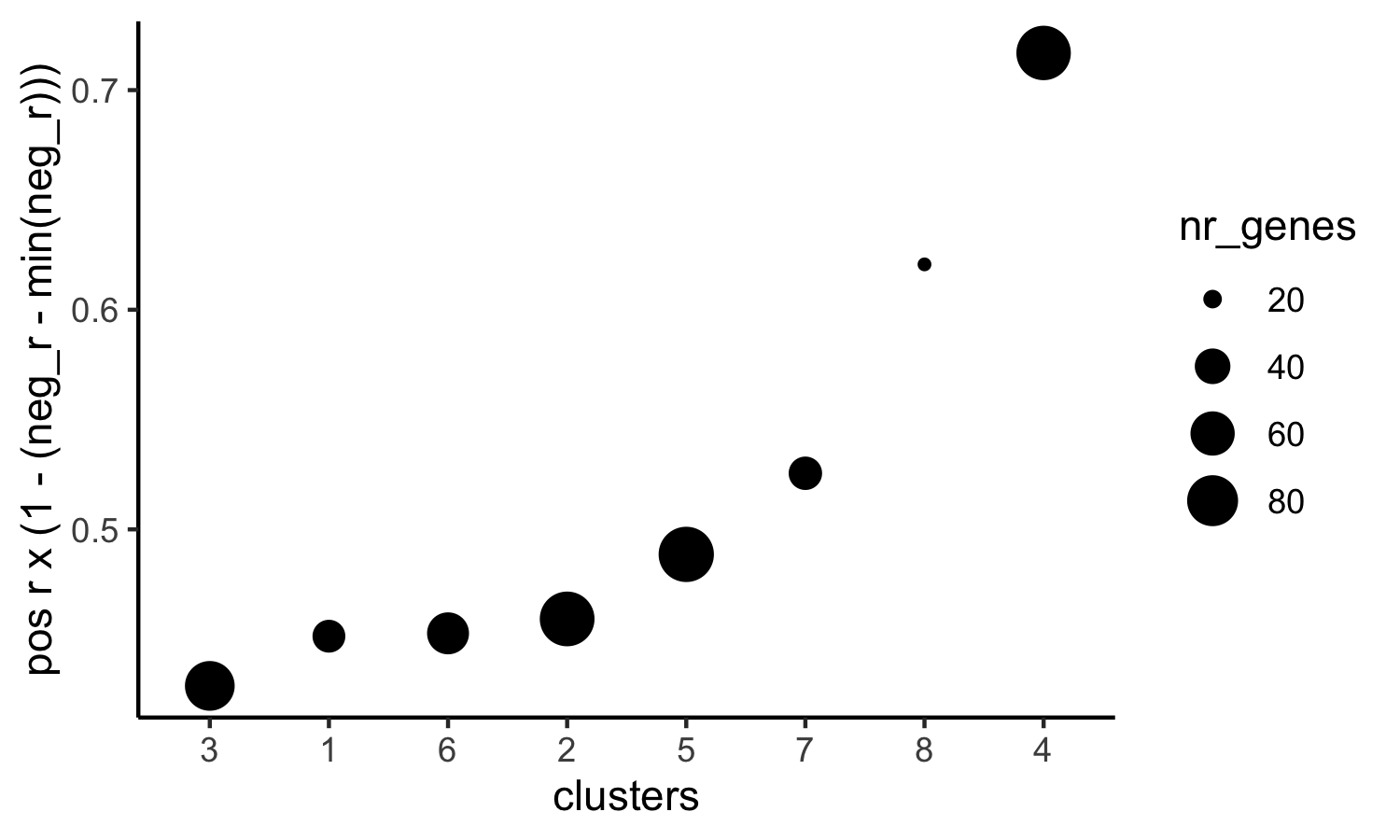top_netw_spat_cluster = showSpatialCorGenes(spat_cor_netw_DT, use_clus_name = 'spat_netw_clus',
selected_clusters = 6, show_top_genes = 1)

# 5. create metagene enrichment score for clusters
cluster_genes_DT = showSpatialCorGenes(spat_cor_netw_DT, use_clus_name = 'spat_netw_clus', show_top_genes = 1)
cluster_genes = cluster_genes_DT$clus; names(cluster_genes) = cluster_genes_DT$gene_ID

visium_kidney = createMetagenes(visium_kidney, gene_clusters = cluster_genes, name = 'cluster_metagene')

spatCellPlot(visium_kidney,
spat_enr_names = 'cluster_metagene',
cell_annotation_values = netw_ranks$clusters, point_size = 1.5, cow_n_col = 4, save_param = c(save_name = '10_g_spat_enrichment_score_plots', base_width = 13, base_height = 6))# example for gene per cluster top_netw_spat_cluster = showSpatialCorGenes(spat_cor_netw_DT, use_clus_name = 'spat_netw_clus', selected_clusters = 1:8, show_top_genes = 1) first_genes = top_netw_spat_cluster[, head(.SD, 1), by = clus]$gene_ID
cluster_names = top_netw_spat_cluster[, head(.SD, 1), by = clus]$clus names(first_genes) = cluster_names first_genes = first_genes[as.character(netw_ranks$clusters)]

spatGenePlot(visium_kidney, genes = first_genes, expression_values = 'scaled', cow_n_col = 4, midpoint = 0, point_size = 2,
save_param = c(save_name = '10_h_spat_enrichment_score_plots_genes',
base_width = 11, base_height = 6))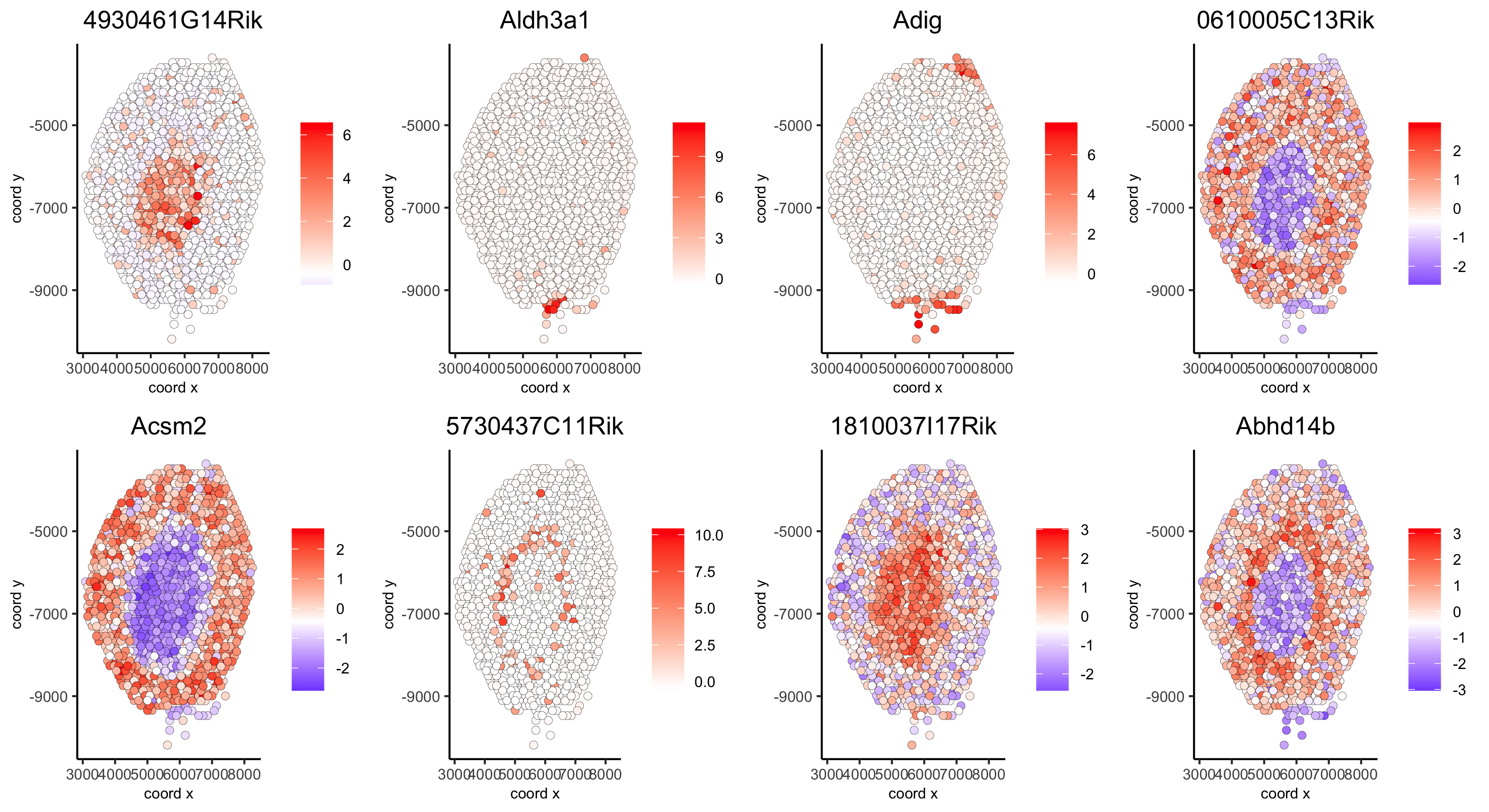## part 11: HMRF domains

# HMRF requires a fully connected network!
visium_kidney = createSpatialNetwork(gobject = visium_kidney, minimum_k = 2, name = 'Delaunay_full')

# spatial genes
my_spatial_genes <- kmtest[1:100]\$genes

# do HMRF with different betas
hmrf_folder = paste0(results_folder,'/','11_HMRF/')
if(!file.exists(hmrf_folder)) dir.create(hmrf_folder, recursive = T)

HMRF_spatial_genes = doHMRF(gobject = visium_kidney, expression_values = 'scaled',
spatial_network_name = 'Delaunay_full',
spatial_genes = my_spatial_genes,
k = 5,
betas = c(0, 1, 6),
output_folder = paste0(hmrf_folder, '/', 'Spatial_genes/SG_topgenes_k5_scaled'))

## view results of HMRF
for(i in seq(0, 5, by = 1)) {
viewHMRFresults2D(gobject = visium_kidney,
HMRFoutput = HMRF_spatial_genes,
k = 5, betas_to_view = i,
point_size = 2)
}
## alternative way to view HMRF results
#results = writeHMRFresults(gobject = ST_test,
#                           HMRFoutput = HMRF_spatial_genes,
#                           k = 5, betas_to_view = seq(0, 25, by = 5))

## add HMRF of interest to giotto object
HMRFoutput = HMRF_spatial_genes,
k = 5, betas_to_add = c(0, 2),
hmrf_name = 'HMRF')

## visualize
spatPlot(gobject = visium_kidney, cell_color = 'HMRF_k5_b.0', point_size = 5,
save_param = c(save_name = '11_a_HMRF_k5_b.0'))
spatPlot(gobject = visium_kidney, cell_color = 'HMRF_k5_b.2', point_size = 5,
save_param = c(save_name = '11_b_HMRF_k5_b.2'))

## Export and create Giotto Viewer

# check which annotations are available

# select annotations, reductions and expression values to view in Giotto Viewer
viewer_folder = paste0(results_folder, '/', 'mouse_visium_kidney_viewer')

exportGiottoViewer(gobject = visium_kidney,
output_directory = viewer_folder,
spat_enr_names = 'PAGE',
factor_annotations = c('in_tissue',
'leiden_clus'),
numeric_annotations = c('nr_genes',
'clus_25'),
dim_reductions = c('tsne', 'umap'),
dim_reduction_names = c('tsne', 'umap'),
expression_values = 'scaled',
expression_rounding = 2,
overwrite_dir = T)